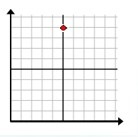# Plotting a point in quadrant 1

#### Complete Python Prime Pack

9 Courses     2 eBooks

#### Artificial Intelligence & Machine Learning Prime Pack

6 Courses     1 eBooks

#### Java Prime Pack

9 Courses     2 eBooks

In this lesson, we learn to plot points in the quadrant 1 given their coordinates.

Consider the following example

Using the pencil, plot the point (4, 5)

### Solution

We move along the horizontal axis or the x-axis four units towards the right from the origin and then move 5 units up vertically to locate the point (4, 5).This is how a point with given coordinates is plotted.

### Rules to plot a point in quadrant 1 given its ordered pair

• We move along the x axis that many units from the origin as the x coordinate.

• Then we move up vertically as many units as the y coordinate to locate the point in the quadrant 1.

Plot the following point in the quadrant 1

(5, 9)

### Solution

Step 1 − The x and y coordinates of the point are 5 and 9 respectively.

Step 2 − We move five units from the origin to the right on the x axis and then move up vertically 9 units to locate the given point.

Step 3 − Plotting the given point in the quadrant 1 we get the followingPlot the following point in the quadrant 1

(6, 8)

### Solution

Step 1 − The x and y coordinates of the point are 6 and 8 respectively.

Step 2 − We move 6 units from the origin to the right on x axis and then move vertically 8 units to locate the given point.

Step 3 − Plotting the given point in the quadrant 1 we get the following